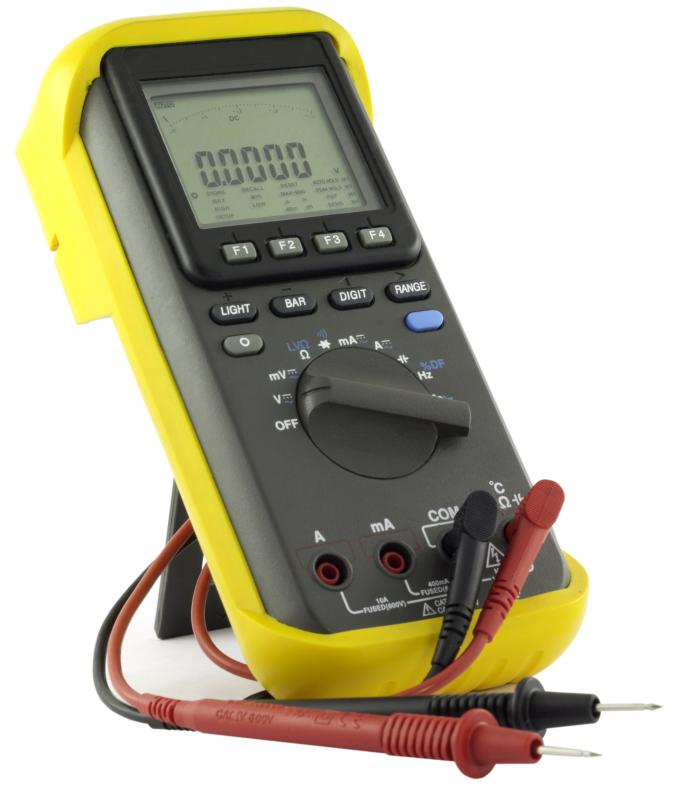# What Are Volt-Amperes?

Andrew Kirmayer

Electrical power is often measured in volts, as well as amperes which are a measure of the current. Volt-amperes (VA), sometimes referred to as apparent power, is a value determined by multiplying the voltage of a piece of electrical equipment by the current it draws. These units can help to determine what kind of power supply or circuit breaker is needed for electrical equipment such as a computer. Watts, in contrast, represent the actual power being drawn by the system. Typically used with direct current (DC) circuits, volt-amperes are sometimes calculated for alternating current (AC) systems as well.A multimeter, which can be used to measure voltage and amps.

Volt-amperes generally reflect the power used by a DC circuit. In AC circuits, inductors and capacitors alter the electrical current by producing reactance, which usually results in a higher VA rating than the wattage. The power expressed in watts is typically one-half to two-thirds of the volt-ampere rating in an AC system. Selecting equipment and accompanying universal power supplies often requires this knowledge to find products with the proper specifications.

The rating in volt-amperes can typically be calculated by multiplying the wattage of a system by 1.67. This formula is often useful for determining the power needs of computers and related equipment, such as monitors, printers, and various other peripherals that draw power. The power supply’s capacity can be derived from multiplying its VA rating by 60 percent, so both the supply and the equipment can be accurately assessed. Power supplies should generally have a higher rating than the equipment, which can help to avoid problems if an electrical surge occurs.

Another use of VA specifications is often the measurement of reactive power. Reactive power is typically needed in capacitors and inductors of circuits to create electric and magnetic fields. Measured in volt-amperes-reactive, it is often important for power transmission lines. High current flows with reactive power can result in electrical losses, which are often troublesome to power transmission systems.

For an incandescent light bulb, volt-amperes and watt ratings usually mean the same thing. The two rating systems, however, are different for most other electrical devices. Computer power supplies often have a power factor correction system that makes the watt and VA ratings equal. How close the two ratings match are determined by a number less than or equal to one. A power factor of one generally means the ratings for wattage and volt-amperes are the same; the measurement is often used for servers as well as switches and routers.

## You might also Like